## What is a Support Vector Machine?

The idea of a Support Vector Machine (SVM) is simple: To separate two clusters of points using a line, as you can see in the following image. The black line is separating cluster B from cluster A: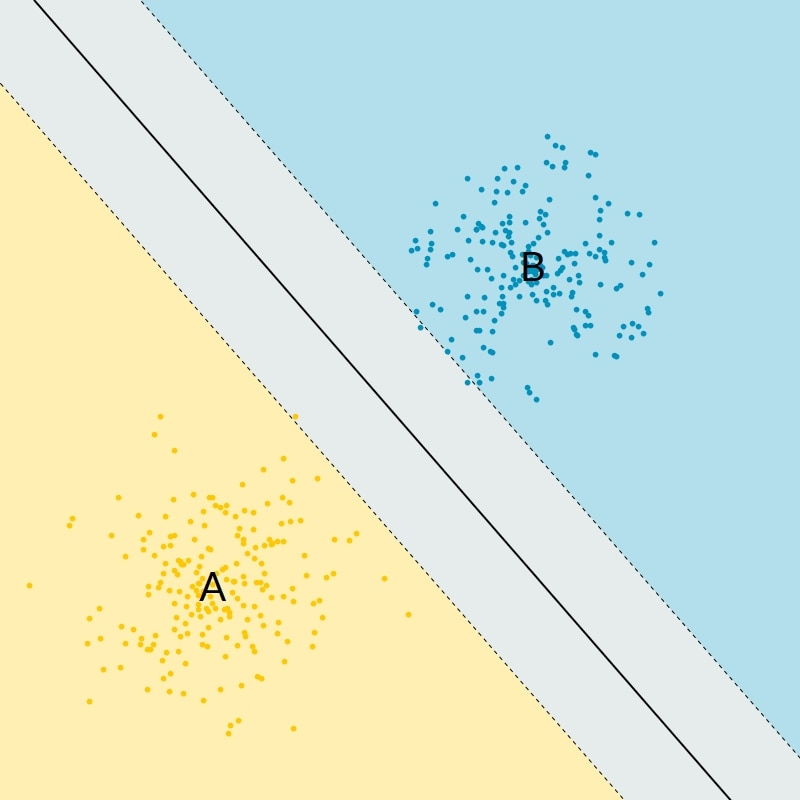Figure 1: Simple linear SVM classifier

The SVM classifier is a binary classifier. There are only two classes. In practice the points are not often points in a plane. Instead the points are feature vectors in a higher-dimensional space and therefore the line is a hyperplane.

Also, there is no reason for the two clusters of points to be separable with a hyperplane, as you can see in the following image: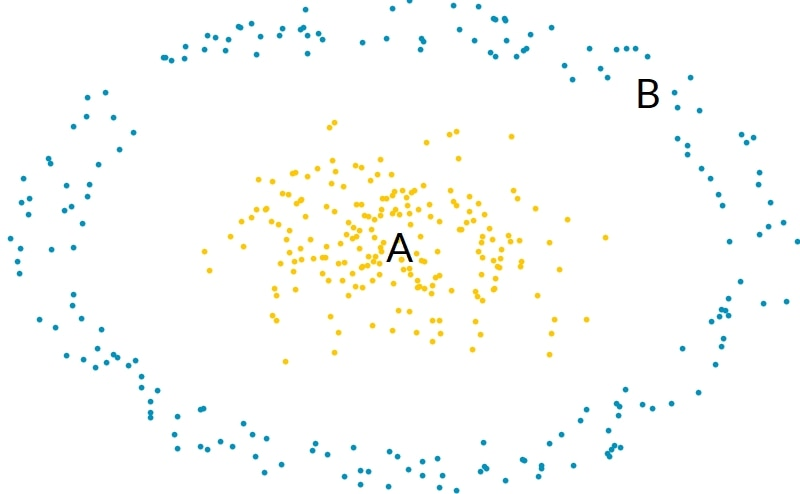Figure 2: Two clusters of points: Cluster A and Cluster B

Cluster A and B cannot be separated by a plane. To solve this issue, SVM algorithms introduce nonlinear transformations.

In CMSIS-DSP, four kinds of transformations are supported and therefore four kinds of SVM classifiers are available. Those classifiers use vectors, the support vectors, and coefficients, named dual coefficients, which are generated by the training process.

### The linear classifier

The linear prediction uses the following formula: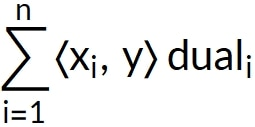Support vectors xi and dual coefficients are generated during the training. The vector to be classified is y. <x,y> is the scalar product between vectors x and y.

The sign of this expression is used to classify the vector y as belonging to class A or B.

### Polynomial classifier

The polynomial classifier uses the following formula: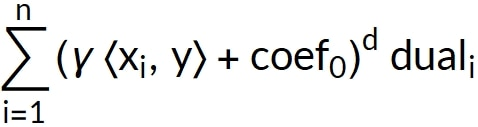This formula is more complex than the one for the linear classifier. Several new parameters are generated during the training:

• Gamma
• coef0
• The degree d of the polynomial

The radial basis function classifier uses the following formula: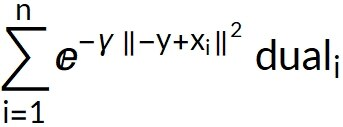Instead of using a scalar product, Euclidean norm is used. A radial basis function is a function with a value that depends on the distance to a fixed reference point: In this case, the distance between xi and the value to be classified y.

### Sigmoid

The sigmoid classifier uses the following formula: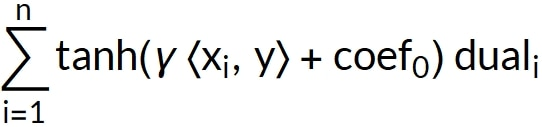This formula is like the polynomial formula, but instead of computing the power of the expression, tanh is used.

Because the polynomial SVM is the SVM classifier that requires the most parameters, we use the polynomial SVM as an example in this guide. You will learn how to train the polynomial classifier in Python and how to dump the parameters to use the trained classifier in CMSIS-DSP.